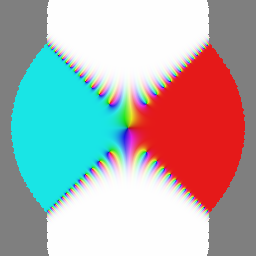# Hypergeometric functions in Arb

November 9, 2014

The git master of Arb now supports evaluating complex-valued hypergeometric functions. Currently, the following can be done:

• Evaluating the generalized hypergeometric function $${}_pF_q(\mathbf{a}, \mathbf{b}, z) = \sum_{k=0}^\infty \frac{(a_1)_k\dots(a_p)_k}{(b_1)_k\dots(b_q)_k} \frac {z^k} {k!}$$ for arbitrary complex inputs when the series converges, with rigorous error bounds.
• Evaluating the confluent hypergeometric function $U(a,b,z)$ (and thus indirectly also ${}_0F_1(b,z)$ and ${}_1F_1(a,b,z)$) for complex $a, b, z$ using asymptotic expansions with respect to $z$, with rigorous error bounds.
• Evaluating two particular special functions implemented using hypergeometric functions: the error function $\operatorname{erf}(z)$ and the Bessel function of the first kind $J_{\nu}(z)$, for any $\nu, z \in \mathbb{C}$, complete with use of asymptotic expansions when $|z|$ is large.

To have something pretty to show, here is a plot of $J_{-1-4i}(z)$ as a function of $z$ on $[-10,10] + [-10,10] i$, and a plot of $J_{\nu}(-1-4i)$ as a function of $\nu$ (see this earlier post for the plotting code):Before you get too excited, please note that the following important features (all found in mpmath, but of course without error bounds) are not available:

• Analytic continuation of ${}_2F_1$, ${}_3F_2$, etc. and evaluation of these functions close to the singular point $z = 1$.
• Asymptotic expansions of higher order hypergeometric functions.
• Evaluation of parameter limits (needed to compute $K_n(z)$ when $n \in \mathbb{Z}$, for example).
• Borel summation of divergent series.

Also, no asymptotically fast algorithms (binary splitting, bit-burst evaluation, summation using fast multipoint evaluation) have been implemented yet. But note that Arb already does feature binary splitting evaluation of hypergeometric series with purely rational coefficients, used for high-precision computation of constants such as $\pi$.

As mentioned above, I've already implemented the error function and the first Bessel function. Other functions that can be implemented in a similar way using the available tools include: exponential/trigonometric/logarithmic integrals, various scaled error functions, the Bessel function $I_{\nu}(z)$, Airy functions, upper and lower incomplete gamma functions, and a few others. Some other hypergeometric special functions can not be implemented as straightforwardly: $K_n(z)$ is an example; another is the Legendre function $P_{\nu}(z)$ for noninteger $\nu$, which requires analytic continuation of ${}_2F_1$ unless $|z| \ll 1$.

The mandatory benchmark shootout is between MPFR 3.1.2 (real arguments only), mpmath in Sage-6.3, and Arb. Here are timings in seconds for computing $\operatorname{erf}(z)$:

 $z$ Digits MPFR mpmath Arb $\pi$ 10 0.000021 0.00011 0.000030 $\pi$ 100 0.00020 0.00033 0.00011 $\pi$ 1000 0.012 0.0048 0.0014 $\pi$ 10000 1.3 0.76 0.071 $10 \pi$ 10 0.00000023 0.000011 0.0000062 $10 \pi$ 100 0.00000026 0.000011 0.0000061 $10 \pi$ 1000 0.089 0.061 0.0040 $10 \pi$ 10000 4.1 1.7 0.15 $(10+10i) \pi$ 10 - 0.00036 0.000013 $(10+10i) \pi$ 100 - 0.00042 0.000070 $(10+10i) \pi$ 1000 - 0.10 0.019 $(10+10i) \pi$ 10000 - 17 0.26

Unsurprisingly, MPFR is faster at low precision and when the result is indistinguishable from 1, since it uses non-generic code with less overhead. Arb is faster at higher precision mainly due to using rectangular splitting for series summation.

I did cheat a bit with the complex input $z = (10+10i) \pi$ in this benchmark. The way the error function is implemented right now, it computes with the same internal precision as the target precision. At 10 and 100 digits, the asymptotic expansion is used for this input, which gives full accuracy. But the asymptotic expansion only gives up to 850 digit accuracy for this input. So at 1000 and 10000 digits, the convergent ${}_1F_1$ series is used. However, this loses some 850 digits due to cancellation in the summation. So for this benchmark, I increased the precision by 3000 bits to get timings for actually computing a result accurate to 1000 and 10000 digits respectively. The code could be improved to automatically estimate the amount of cancellation and adjust the precision accordingly. This is only a problem near the "diagonals" in the complex plane: near the imaginary and real axes, erf is computed using cancellation-free series.

Here is a plot of erf on $[-10,10] + [-10,10] i$. To generate it, I edited the plotting code to automatically repeat with higher precision on any point near the diagonal where the output turned out have poor accuracy at the initial precision of 128 bits. The possibility to easily detect and correct such errors is a luxury that comes with ball or interval arithmetic!Below are three plots generated only using a single algorithm and a precision of 128 bits, with a gray pixel inserted whenever this failed to give more than 3 bits of accuracy. Left: the ${}_1F_1$ series, middle: the ${}_1F_1$ series with Kummer's transformation, right: the asymptotic series.To conclude, here are timings for computing the Bessel function $J_{\nu}(z)$. MPFR only supports integer $\nu$ and real $z$.

 $\nu$ $z$ Digits MPFR mpmath Arb 2 $\pi$ 10 0.0000058 0.00017 0.0000098 2 $\pi$ 100 0.000037 0.00027 0.000046 2 $\pi$ 1000 0.0022 0.0020 0.00060 2 $\pi$ 10000 0.41 0.33 0.032 2 $10^6 \pi$ 10 0.000013 0.00040 0.000014 2 $10^6 \pi$ 100 0.000064 0.00036 0.000073 2 $10^6 \pi$ 1000 0.0054 0.012 0.0020 2 $10^6 \pi$ 10000 0.64 1.1 0.19 2+3i $(10+10i) \pi$ 10 - 0.00080 0.000045 2+3i $(10+10i) \pi$ 100 - 0.0022 0.00033 2+3i $(10+10i) \pi$ 1000 - 0.95 0.014 2+3i $(10+10i) \pi$ 10000 - - 3.8 $(1+i)\pi$ $(100+100i) \pi$ 10 - 0.00075 0.000042 $(1+i)\pi$ $(100+100i) \pi$ 100 - 0.0019 0.00063 $(1+i)\pi$ $(100+100i) \pi$ 1000 - 0.64 0.079 $(1+i)\pi$ $(100+100i) \pi$ 10000 - - 12.2